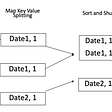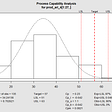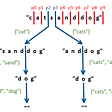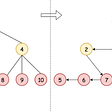# Problems With Advanced DS — Sorted Sets

In this post we are going to look at data structures for storing stream of numbers in a way such that the following operations can be efficiently performed (although the data structures we are going to visit here can do a lot more):

1. Current minimum and maximum numbers.
2. Median of all numbers seen so far.
3. Smallest (Largest) number greater (smaller) than a given number.
4. Count of all smaller (or larger) numbers given a number.
5. Binary search on numbers seen so far.

For 1 and 2, we can use a Min-Heap and a Max-Heap. 3 and 4 are special cases of Binary Search (5). But all of the above use cases can be solved efficiently if the numbers are sorted.

But the problem lies in the fact that the numbers are in an infinite stream and thus for each incoming number we have to sort the entire list of numbers seen so far. That is very inefficient space and time complexity wise as we do not know when/where the stream will end.

There are various data structures that can solve all of the above problems in O(logN) time complexity.

But there is one problem. All of the above data structures are quite complicated implementation wise (what I mean here is that coding any one of them would take up all your 45 minutes-1 hour coding interview time).

For most problems that require sorted stream of numbers, the particular use case can be solved efficiently using some other strategy instead of sorted sets data structure. Knowing the tricks is helpful from interviewing perspective.

In this post we will solve problems using non-sorted set tricks that can also be solved using sorted sets data structures mentioned above.

Problem 1:

Count of Smaller Numbers After Self — You are given an integer array nums and you have to return a new counts array. The counts array has the property where `counts[i]` is the number of smaller elements to the right of `nums[i]`.

Assume that the range of numbers is 10^-4 to 10⁴

Solution:

This is a simple case of self balancing BST. Starting from the end of the array, we insert the numbers into the self balancing BST. Keep node attributes ‘left_count’ and ‘right_count’ with each node. Then for each ‘nums[i]’, whenever we go to right of a node during insertion (‘nums[i] > root.val’) then increment smaller_count by (root.left_count+1).

AVL Tree implementation is as follows:

Just look at the amount of code required for AVL Tree and that is only the insertion part (for this problem, only insertion into AVL Tree is required).

The time complexity with AVL Tree is O(N*logN).

The same problem can be efficiently solved using a Segment Tree by observing that the range of values for nums[i] is O(10⁴).

Create a segment tree for the range [min(nums[i]), max(nums[i])]. Then for each nums[i], insert nums[i] into the segment tree by incrementing the counter at nums[i]. To count number of smaller numbers for a given number, at each index ‘i’, compute the range sum for [0, nums[i]-1] (i.e. number of values less than nums[i]) using the segment tree.

Time complexity for segment tree approach is O(M*logM) where M=(max(nums)-min(nums)).

But the amount of code required for the segment tree is relatively shorter than AVL Tree. As for the running time, segment tree code runs almost 90% faster than the AVL Tree implementation.

But note that if the range for nums would have been say O(10⁹) then segment tree implementation would be inefficient. For smaller range numbers, segment tree solution is most efficient.

One way we can overcome the limitations of the segment tree for large range numbers is by mapping the N numbers in the array to a space of size N. For example, sort the N numbers in the array, then starting from the 0-th index, map the smallest numbers to 0, the second smallest to 1 and so on. In this way we would have mapped to at-most a range of [0, N-1].

E.g. if nums=[21, 67, 19076, 1, 954, 33, 67, 21, 1000000000]

The after sorting [1, 21, 21, 33, 67, 67, 954, 19076, 1000000000]. After mapping we would obtain [0, 1, 1, 2, 3, 3, 4, 5, 6]. Now the range has ben transformed from [1, 1000000000] to [0, 6]

new_nums = [1, 3, 5, 0, 4, 2, 3, 1, 6].

With the above modification, approach with segment tree also becomes O(N*logN)

But wait, there is still a better approach: Using merge sort.

Do merge sort on the array. But before doing merge operation on 2 sorted arrays A and B, we can count the number of smaller numbers in B for each A as follows:

Assuming that we are sorting in increasing order, start from the last index in A and last index in B. If A[i] ≤ B[j], then decrease j by 1. Else if A[i] > B[j] then increment the count of smaller numbers for A[i] by (j+1) and decrease i by 1. Continue until either i < 0 or j < 0.

During each level of recursion, we will be updating the smaller counts for a particular index from all previous levels of the recursion.

Run time complexity of the above approach is still O(N*logN) but it runs more than 100% faster than segment tree approach.

Problem 2:

Reverse Pairs — Given an array `nums`, we call `(i, j)` an important reverse pair if `i < j`and `nums[i] > 2*nums[j]`.

You need to return the number of important reverse pairs in the given array.

Solution:

The problem is similar to the above problem. We can use a AVL Tree to solve it efficiently.

For example, starting from 0, we index the numbers into AVL Tree. To count reverse pairs, before we insert nums[i], lookup in the AVL Tree for all nodes with root.val > 2*nums[i].

If root.val ≤ 2*nums[i], then go towards right. If root.val > 2*nums[i] then increment counter by (root.right_count+1) and go left.

Not showing the entire code, but we need to add this function to the AVLTree class and call it to get the number of reverse pairs for a given index.

The problem can be solved efficiently again using Segment Tree.

One approach is same as the one shown in the last problem. We map the values in the input array to [0, N-1] range and then for each i, count the range sum of [f[2*nums[i]+1], N-1] where f[x] finds the smallest number greater than equals to ‘x’ in the array ‘nums[i]’ and then apply the above map to project it to a number in the range [0, N-1]. This involves quite a lot of steps.

Another approach is to create the list of tuples H=[(nums[i], i)], sort this list on the 1st value i.e. nums[i] in decreasing order and then at each index ‘j’ in H insert H[j] into the segment tree.

Basically we go from 0-th index in H and also keep another iterator ‘j’ starting from index 0. If at index ‘i’ in H, H[j] > 2*H[i], then add H[j] to segment tree and increment ‘j’. Do this until H[j] ≤ 2*H[i]. Then increment ‘i’ and continue again with the same steps.

Time complexity of both AVL Tree and Segment Tree is O(N*logN).

But there is an even better approach using Divide and Conquer strategy (with the same run-time complexity).

Do merge sort on the array. But while doing merge operation on 2 sorted arrays A and B, we can count and store the number of reverse pairs in the combined array A+B.

Let A be the sorted 1st half of the array and B be the sorted 2nd half of the array (A+B). Then start from the 0-th index in A and 0-th index in B. If A[i] ≤ 2*B[j], then increment i by 1. Else if A[i] > 2*B[j], then increment count = count + (len(A)-i) and increment j by 1. Continue like this.

During each level of recursion, we will keep adding all the reverse pairs from all previous levels of the recursion.

Problem 3:

Find Median from Data Stream — Design a data structure that supports the following two operations:

• void addNum(int num) — Add a integer number from the data stream to the data structure.
• double findMedian() — Return the median of all elements so far.

Solution:

This is again an application of AVL Tree. In ‘addNum’ function, we insert ‘num’ into AVL tree. In ‘findMedian’ function, we can find median by following the steps below (Assuming we store left_count and right_count with each node):

Let a=Count of smaller numbers than current root, b=Count of larger numbers than current root

1. If (a + root.left_count == b + root.right_count) then return root.val
2. If (a + root.left_count) — (b + root.right_count) == 1, then find the previous smaller number to root.val by getting the rightmost node ‘temp’ of the left subtree. median = (root.val+temp.val)/2.0
3. If (a + root.left_count) — (b + root.right_count) == -1, then find the next higher number to root.val by getting the leftmost node ‘temp’ of the right subtree. median = (root.val+temp.val)/2.0
4. If (a + root.left_count) — (b + root.right_count) > 1, then increment b by 1+root.right_count and go left recursively i.e. root=root.left
5. If (a + root.left_count) — (b + root.right_count) < -1, then increment a by 1+root.left_count and go right recursively i.e. root=root.right

Both insertion and findMedian lookup is O(logN) with the above approach.

But there is a better approach: Using a min and a max-heap.

If we have seen N numbers in the stream so far, then store the highest N/2 numbers in the min-heap and the smallest N/2 numbers in a max-heap.

If N is even then the median is the average of the root nodes of min-heap and max-heap. Else if N is odd then median is the root node of min-heap.

Whenever a new number comes, if it is smaller than the maximum of the smaller half, then this number gets inserted into the max-heap. If the size of max-heap exceeds N/2 then move the highest i.e. root to min-heap.

Similarly if the new number is greater than the minimum of the greater half, then this number gets inserted into the min-heap. If the size of min-heap exceeds N/2 then move the smallest i.e. root to max-heap.

Time complexity for ‘addNum’ is O(logN) but O(1) for ‘findMedian’.

Problem 4:

Count of Range Sum — Given an integer array `nums`, return the number of range sums that lie in `[lower, upper]` inclusive.

Range sum `S(i, j)` is defined as the sum of the elements in `nums`between indices `i` and `j` (`i``j`), inclusive.

Solution:

One trivial solution is using AVL Tree.

We need to find at each index ‘i’ what are the number of range sums in the range [lower, upper] starting at index ‘i’ ?

If we compute the suffix sums starting from the end of the array and store the suffix sums in a AVL Tree, then at index ‘i’ if the suffix sum is S, we need to find how many suffix sums are there in the tree within the range [S-upper, S-lower] (because lower ≤ upper implies S-upper ≤ S-lower)

In the AVL Tree, if we store ‘left_count’ and ‘right_count’ with each node then if we find :

a = Number of nodes with value greater than equal to S-upper

b = Number of nodes with value greater than equal to S-lower+1

Then ‘a-b’ gives the number of range sums in the range [lower, upper] starting at index ‘i’

Time complexity of the above approach is O(N*logN).

But we can improve the code size and readability by using segment tree approach which we have seen earlier and also merge sort based approach. Since merge sort based approach is the most concise, I will show that code here:

Compute the merge sort steps on the suffix sum array.

Basically before the merge step, given the sorted ‘suffix sum arrays’ A and B, for each A[i] find all numbers in B where A[i]-upper ≤ B[j] ≤ A[i]-lower. This can be done using either binary search (which is O(N*logN)) or better using a double ended queue (O(N)).

Thus the time complexity of the merge sort based approach is still O(N*logN) but the code much shorter and faster.

Problem 5:

Sliding Window Maximum — You are given an array of integers `nums`, there is a sliding window of size `k`which is moving from the very left of the array to the very right. You can only see the `k` numbers in the window. Each time the sliding window moves right by one position.

Return the max sliding window.

Solution:

One solution is to use an AVL Tree. For the 1st sliding window, insert the ‘k’ numbers into AVL Tree. Maximum number can be found in O(logK) time complexity. When the window is moved to the right, delete the 1st number (index 0) from the AVL Tree and insert the new number (index k). Deletion and insertion is again O(logK).

Thus time complexity of the above approach is O(N*logK).

Another approach is to use a heap. In the first sliding window, insert all the numbers into a max-heap and in each subsequent window insert the new number into a max-heap. To find the maximum number we return the root of the heap.

Since it is not straightforward to delete a number other than the root in a heap, thus instead of deleting the 1st number in the sliding window when we move the window to right, we delete the root of the max-heap if the index of the root is less than the start index of the current sliding window.

Since the maximum size of the heap at any point in time is O(N). Thus time complexity of the above approach is O(N*logN) (when the array is sorted in increasing order).

There is even a better approach using O(N) time complexity using double ended queue.

At any point in time, maintain a decreasing order sequence of numbers in a deque. Then the maximum in the current window is always the first number in the queue.

That’s all in this post. What we conclude is that most sorted set problems that require self balancing BST (AVL Tree etc.) can be solved using some or the other tricks which can be useful during interviews.

In the next post we will look at problems involving Tries.

--

--

--

## More from The Startup

Get smarter at building your thing. Follow to join The Startup’s +8 million monthly readers & +756K followers.

## LIGHTNIFT NFT DATATHON: FOR A SUSTAINABLE NFTverse## A simple guide to understand PCA## Optimising Knockout Rules for Lending## Analytics of FHV ridership dataset(NYU CSCI-476 Extra Credit)## Budgeting based on process capability## Trust Region and Proximal Policy Optimization## Getting started with Kaggle using Facial Detection## Future big events prediction with: how to build your own Crystal ball using public tweets and LSTM…Engineer

## Flexible Health IT Models: The use of custom data structures## Leetcode 140. Word Break II — Solution Explanation## 431. Encode N-ary Tree to Binary Tree## Product of Array Except Self